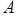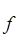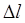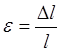Fundamentals

Tensile, Compressive and Bending Stresses and Strains

Stress represents the action of a force or moment on a structural member. If the force pulls the member (tension) it results in a tensile stress; if the force pushes the member (compression) it results in compressive stress. Tensile stresses stretch a member and compressive stresses squeeze a member.

There is a significant difference between the behavior of a structural member in tension and compression. Depending on how slender the structural member is, it may buckle or crush under compression stresses. However, buckling does not occur when a structural member is subjected to tensile stresses. Stress () is defined as force () divided by area ():has units of  lb/in2 (psi) or k/in2 (ksi)
 Compression Stress Distribution
Tensile stress results in the elongation of the member. If the original length is (l) and the change in the length is, strain () is defined as:Therefore, stress represents the applied force and strain represents the resulting deformation.

Tensile Stress Distribution

Bending is due to the internal moment. Since moment can be resolved into a couple, the internal moment can be considered as a compression force (C) and a tensile force (T). The compression force results in compressive stresses and tensile force in tensile stresses. Therefore, bending stress is a combination of compressive and tensile stresses due to  internal moments.

Since the stress across a beam section varies from compression to tension, there is a location at which stress is equal to zero. This is called the “neutral axis”. For a homogeneous beam the neutral axis passes through its centroid.

Bending Stress Distribution© 2011 Virginia Polytechnic Institute and State University